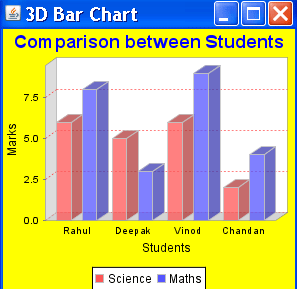Tutorials

3D Bar Chart

In this section we are providing you an example to create a 3D Bar Chart.

In this section we are providing you an example to create a 3D Bar Chart.

3D Bar Chart

In this section we are providing you an example to create a 3D Bar Chart.

Description of Program

For creating a 3D Bar chart we use the object of DefaultCategoryDataset. After creating the object of DefaultCategoryDataset we add some data in this object by invoking setValue() method. In this example you learn some more interactive features of JFreeChart like set the background color of chart and adjust the color the title etc.

Description of Code

For defining a dataset for a Bar chart we have to create an object of DefaultCategoryDataset type :
DefaultCategoryDataset dataset = new DefaultCategoryDataset();

After creating the instance of dataset then we have to add the data in the data set by invoking the method setValue(). In this example we show more than one set of bars for the same chart. This can done by the following modification :
dataset.setValue(6, "Science", "Rahul");
dataset.setValue(8, "Maths", "Rahul");
dataset.setValue(5, "Science", "Deepak");
dataset.setValue(3, "Maths", "Deepak");

First argument specifies the total marks obtained by the student and the second argument specify what will appear in the legend to the meaning of the bar.

JFreeChart chart = ChartFactory.createBarChart3D("Comparison between Students", "Student", "Marks", dataset, PlotOrientation.VERTICAL, true, true, false);
After added the data in dataset we create the 3D Bar chart by invoking the createBarChart3D() method. This method is a static method of ChartFactory class and its returns the object of JFreeChart type.This method syntax is:

Public static JFreeChart createBarChart3D(java.lang.String title, java.lang.String categoryAxisLabel, java.lang.String valueAxisLabel, CategoryDataset dataset, PlotOrientation orientation, boolean legend, boolean tooltips, boolean urls);

chart.setBackgroundPaint(Color.yellow);
Above method is used to set the color of chart background

chart.getTitle().setPaint(Color.blue);
Above method is used to set the color of chart title

CategoryPlot p = chart.getCategoryPlot();
Above method is used to get the object of the Plot for Bar Chart

p.setRangeGridlinePaint(Color.red);
Above method is used to set the color of plot Gridlines

ChartFrame frame1=new ChartFrame("Bar Chart",chart);
After this we create the object of ChartFrame. It used to display a chart.

Here is code of Program :

 import org.jfree.chart.*; import org.jfree.data.category.*; import org.jfree.data.general.DefaultPieDataset; import org.jfree.data.xy.*; import org.jfree.data.*; import org.jfree.chart.renderer.category.*; import org.jfree.chart.plot.*; import java.awt.*; public class BarExample2{ public static void main(String arg[]){   DefaultCategoryDataset dataset = new DefaultCategoryDataset();   dataset.setValue(6, "Science", "Rahul");   dataset.setValue(8, "Maths", "Rahul");   dataset.setValue(5, "Science", "Deepak");   dataset.setValue(3, "Maths", "Deepak");   dataset.setValue(6, "Science", "Vinod");   dataset.setValue(9, "Maths", "Vinod");   dataset.setValue(2, "Science", "Chandan");   dataset.setValue(4, "Maths", "Chandan");   JFreeChart chart = ChartFactory.createBarChart3D   ("Comparison between Students","Students", "Marks",    dataset, PlotOrientation.VERTICAL, true,true, false);   chart.setBackgroundPaint(Color.yellow);   chart.getTitle().setPaint(Color.blue);    CategoryPlot p = chart.getCategoryPlot();    p.setRangeGridlinePaint(Color.red);    ChartFrame frame1=new ChartFrame("3D Bar Chart",chart);   frame1.setVisible(true);   frame1.setSize(300,300);   } }

Output of the Program :